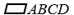Chapter 4.1, Problem 10EElementary Geometry For College St...

7th Edition
Alexander + 2 others
ISBN: 9781337614085

Solutions

Chapter
SectionElementary Geometry For College St...

7th Edition
Alexander + 2 others
ISBN: 9781337614085
Textbook Problem

Given that m ∠ A = 2 x + 3 , and m ∠ C = 3 x - 27 , find the measure of each angle of.To determine

To find:

The measure of each angle of ABCD.

Explanation

Given:

Given that mA=2x+3, and mC=3x-27, and the ABCD as shown below.

Corollary:

1. The opposite angles of a parallelogram are congruent.

2. Two consecutive angles of a parallelogram are supplementary.

In the given parallelogram ABCD, given that mA=2x+3, and mC=3x-27.

As per the corollary that the opposite angles of a parallelogram are congruent, the opposite angles mA and mC are equal or mA=mC and also mB and mD are equal or mB=mD.

To find the value of x, substitute mA=2x+3, and mC=3x-27 in mA=mC

2x+3=3x-27

2x-3x=-27-3

-x=-30

x=30

To find mA, substitute x =30 in mA=

Still sussing out bartleby?

Check out a sample textbook solution.

See a sample solution

The Solution to Your Study Problems

Bartleby provides explanations to thousands of textbook problems written by our experts, many with advanced degrees!

Get Started

Find the mean, median, and mode for the following scores; 8, 7, 5, 7, 0, 10, 2, 4, 11, 7, 8, 7

Essentials of Statistics for The Behavioral Sciences (MindTap Course List)

Prove the statement using the , definition of a limit. limx2(x2+2x7)=1

Single Variable Calculus: Early Transcendentals, Volume I

Simplify x3+x2. 5x6 5x12 2x5 x5

Trigonometry (MindTap Course List)

An integral for the solid obtained by rotating the region at the right about the y-axis is:

Study Guide for Stewart's Single Variable Calculus: Early Transcendentals, 8th

True or False: Du f(a, b) = ∇(a, b) · u.

Study Guide for Stewart's Multivariable Calculus, 8th